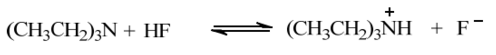# Problem: Does the equilibrium lie to the left or right? (Choose one)

###### FREE Expert Solution
89% (263 ratings)
###### Problem Details

Does the equilibrium lie to the left or right? (Choose one)Frequently Asked Questions

What scientific concept do you need to know in order to solve this problem?

Our tutors have indicated that to solve this problem you will need to apply the Acid Base Equilibrium concept. You can view video lessons to learn Acid Base Equilibrium. Or if you need more Acid Base Equilibrium practice, you can also practice Acid Base Equilibrium practice problems.

What is the difficulty of this problem?

Our tutors rated the difficulty ofDoes the equilibrium lie to the left or right? (Choose one) ...as low difficulty.

How long does this problem take to solve?

Our expert Organic tutor, Chris took 3 minutes and 42 seconds to solve this problem. You can follow their steps in the video explanation above.

What professor is this problem relevant for?

Based on our data, we think this problem is relevant for Professor Hilinski's class at FSU.